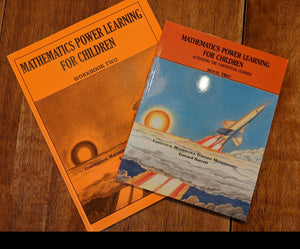Free Shipping on all U.S. Orders!# Level 2 Bundle (3rd-5th)

Professor B

• \$57.00
Unit price per
Shipping calculated at checkout.

Contains Mathematics Power Learning Workbook 2, Mathematics Power Learning Book 2 (3rd-5th).  The Answer Key to Workbook Two is included free as a digital download with this purchase.

### Mathematics Power Learning for Children Level Two consists of two components:

1. Textbook
The textbook for use by the instructor has 160 pages. It contain clear, easy to follow instructions for presenting every lesson and concept. No teacher preparation is needed other than to glance over the lesson before you start. Even older children can teach younger children using this text.

2. Workbook
The workbook offers many unique practice exercises that make it possible for children to achieve the mastery which represents readiness for the next level. The 144-page workbook for Level 2 contains no endless pages of the same question. Purchase one workbook of each level per child. They are NOT reproducible.

### Scope and Sequence of Book 2

Covers: original and effective methods for thorough understanding and mastery of multiplication/division facts, multiplication, long division, fractional equivalence, addition/subtraction of fractions and mixed numbers.
• Counting Numbers
• Multiples
• Factors, prime factorization, finding all factors of a number
• Least common multiples
• Highest common factors
• Mastering the multiplication facts quickly
• Multiplication by large numbers
• Solving word problems with multiplication
• Short division with remainders
• Linking multiplication and division facts
• Long division
• Solving word problems with long division
• Introduction to fractions
• Families of equivalent fractions and reducing to lowest terms
• Adding and subtracting with fractions
• Transforming improper fractions to mixed numbers
• Transforming mixed numbers to improper fractions
• Adding and subtracting with mixed numbers